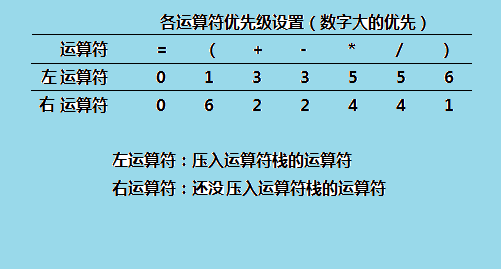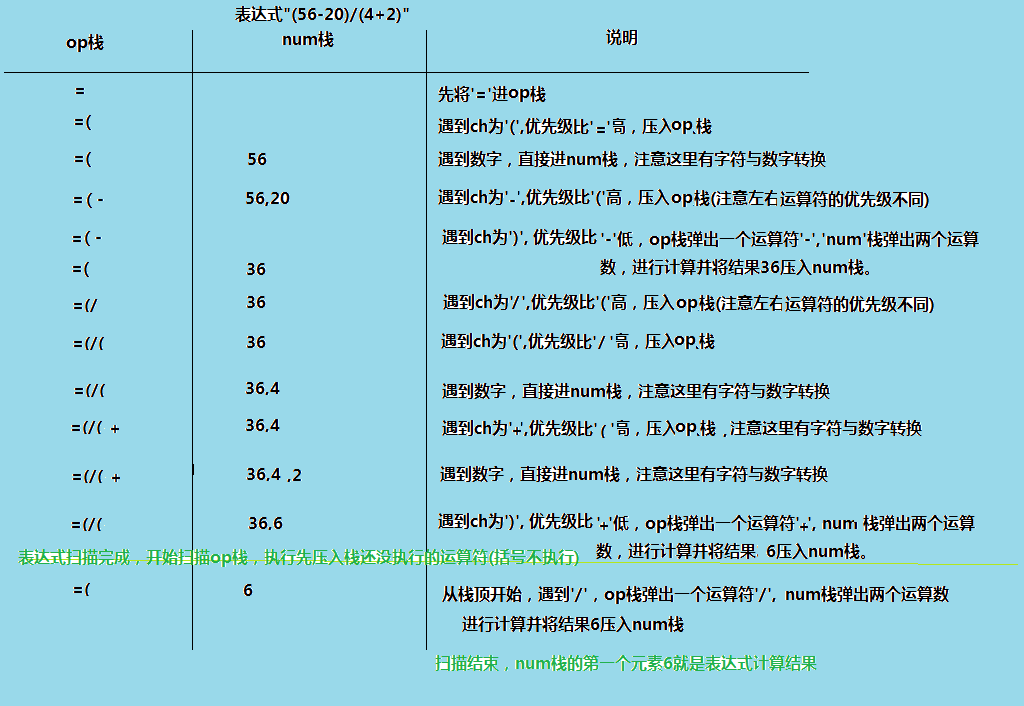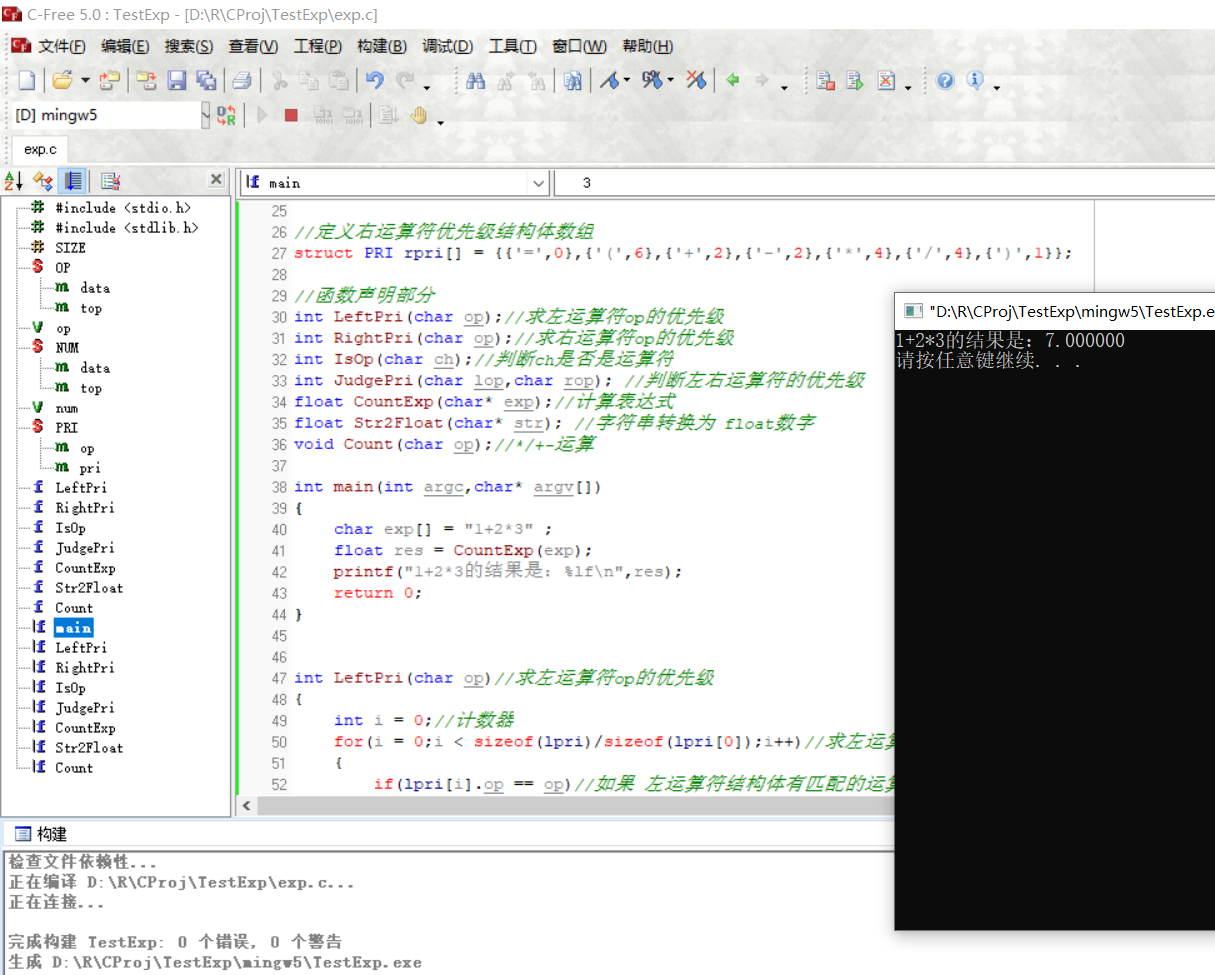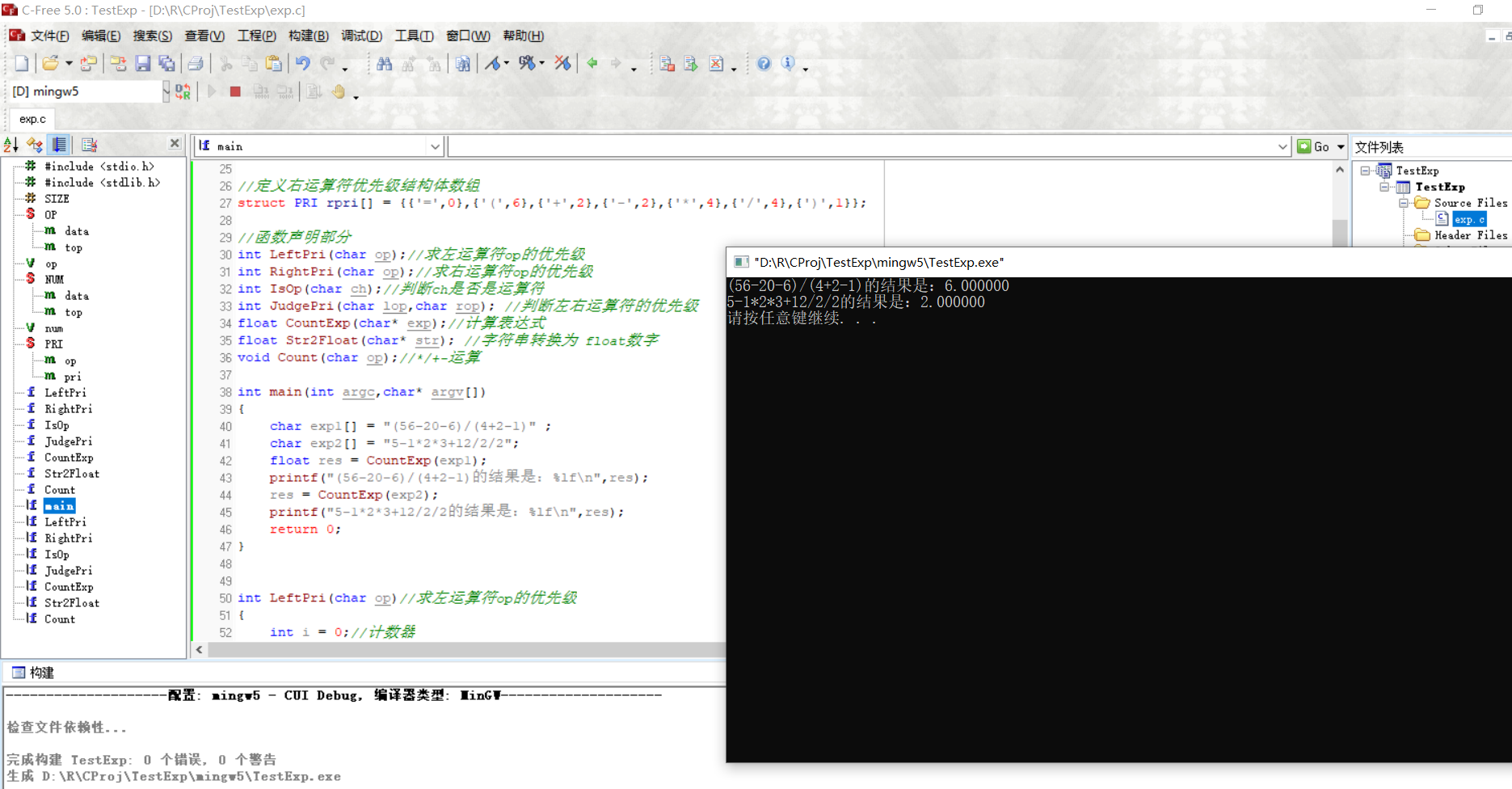• 数据结构与算法计算表达式(一) 一、简单说明 计算中缀表达式。比如1+2*3、（56-20-6）/(4+2-1)。 二、实现主要思路 1、为各运算符指定优先级 说明：‘=’是为了辅助比较运算符，这样子设置优先级，只有...
                                         数据结构与算法  计算表达式(一)

一、简单说明

计算中缀表达式。比如1+2*3、（56-20-6）/(4+2-1)。

二、实现主要思路

1、为各运算符指定优先级说明：‘=’是为了辅助比较运算符，这样子设置优先级，只有括号情况下才会有优先级相等的情况。

2、逐个扫描算数表达式，运算数用一个栈(num)存储、运算符用一个栈(op)存储

3、当右运算符优先级低于左运算符时，op栈弹出一个运算符，num栈弹出两个运算数，然后进行计算。

4、扫描表达式结束，继续扫描执行先压栈而没有执行的运算符

5、最后num栈的第一个元素就是表达式的结果。

举例说明：表达式 (56-20)/(4+2)三、效果四、源码

exp.c源文件

#include <stdio.h>
#include <stdlib.h> //memset()函数所在的头文件
#define SIZE 100   //定义临时存放数据的空间大小

struct  OP //运算符结构体  (这个结构体其实是一个栈的结构)
{
char data[SIZE];         //存放运算符
int top;                 //相当于栈顶指针
}op;                         //定义结构体变量，op存放运算符

struct   NUM//运算数结构体  (这个结构体其实是一个栈的结构)
{
float data[SIZE];         //存放运算数
int top;                 //相当于栈顶指针
}num;                        //定义结构体变量，num存放运算数

struct	PRI		//用来存储运算符的优先级
{
char op;	//运算符
int pri;	//优先级
};

//定义左运算符优先级结构体数组
struct PRI lpri[] = {{'=',0},{'(',1},{'+',3},{'-',3},{'*',5},{'/',5},{')',6}};

//定义右运算符优先级结构体数组
struct PRI rpri[] = {{'=',0},{'(',6},{'+',2},{'-',2},{'*',4},{'/',4},{')',1}};

//函数声明部分
int LeftPri(char op);//求左运算符op的优先级
int RightPri(char op);//求右运算符op的优先级
int IsOp(char ch);//判断ch是否是运算符
int JudgePri(char lop,char rop); //判断左右运算符的优先级
float CountExp(char* exp);//计算表达式
float Str2Float(char* str); //字符串转换为 float数字
void Count(char op);//*/+-运算

int main(int argc,char* argv[])
{
char exp1[] = "(56-20-6)/(4+2-1)" ;
char exp2[] = "5-1*2*3+12/2/2";
float res = CountExp(exp1);
printf("(56-20-6)/(4+2-1)的结果是：%lf\n",res);
res = CountExp(exp2);
printf("5-1*2*3+12/2/2的结果是：%lf\n",res);
return 0;
}

int LeftPri(char op)//求左运算符op的优先级
{
int i = 0;//计数器
for(i = 0;i < sizeof(lpri)/sizeof(lpri);i++)//求左运算符的个数sizeof(lpri)/siozeof(lpri)
{
if(lpri[i].op == op)//如果 左运算符结构体有匹配的运算符
{
return lpri[i].pri;//返回对应的运算符的优先级
}
}

return -1;//没有匹配的运算符
}

int RightPri(char op)//求右运算符op的优先级
{
int i = 0;//计数器
for(i = 0;i < sizeof(rpri)/sizeof(rpri);i++)//求右运算符的个数sizeof(lpri)/siozeof(lpri)
{
if(rpri[i].op == op)//如果 右运算符结构体有匹配的运算符
{
return rpri[i].pri;//返回对应的运算符的优先级
}
}

return -1;//没有匹配的运算符
}

int IsOp(char ch)//判断ch是否是运算符
{
//如果是指定的运算符则返回1，否则返回0
if(ch == '*' || ch == '/' || ch == '+' || ch == '-' || ch == '(' || ch == ')' )
{
return 1;
}
else
{
return 0;
}
}

int JudgePri(char lop,char rop)//判断左右运算符的优先级  左运算符大于右运算符返回1，相等返回0，左小于右返回-1
{
if(LeftPri(lop)> RightPri(rop))//左运算符大于右运算符返回1
{
return 1;
}
else if(LeftPri(lop)== RightPri(rop))//相等返回0，只有左右括号这一种情况
{
return 0;
}
else   //左运算符小于右运算符返回-1
{
return -1;
}
}

float CountExp(char* exp) //计算表表达式
{

memset(&(num.data),0,SIZE);  //将num结构体中的数组data清零
memset(&(op.data),0,SIZE);   //将op结构体中的数组data清零
num.top = -1;                //初始化栈顶指针
op.top= -1;

op.data[++op.top] = '=';  //将'='进栈 ,先++

int i = 0; //用来指示str位置
char str = {0};//临时存放字符串数字

while(*exp!='\0')    //循环扫描exp表达式
{

if(!IsOp(*exp))   //如果不是运算符
{
i = 0;
memset(str,0,10);//每循环一次次清零
while(*exp>='0' && *exp<='9')//是数字
{
str[i++] = *exp++ ;//将数字字符添加到str中,添加后i加1
}
str[i++] = '#';//人为加上结束符 ，辅助进行字符串转换为 float数字
num.data[++num.top] =  Str2Float(str);//将字符串转换为 float数字并赋值给 num的data数组，注意top先++；
}
else  //是运算符
{
switch(JudgePri(op.data[op.top],*exp))
{
case -1://栈顶的运算符优先级低，进栈
op.data[++op.top] = *exp;
break;
case 0://优先级一样，说明是括号 ，只有这一种情况
op.top--;//将'('退栈
break;
case 1://栈顶的运算符优先级高，先出栈进行计算，后入栈
do
{
Count(op.data[op.top--]);//*/+- 运算
}while(JudgePri(op.data[op.top],*exp) == 1);//直到栈顶的运算符优先级不比 右运算符的优先级高，才准备将右运算符入栈

if(*exp != ')')//如果不是')'才入栈
{
op.data[++op.top] = *exp;
}
break;
default:
break;
}
exp++;//继续向下扫描exp
}

}

for(i = op.top;i>0;i--)//因为上面是按根据表达式执行的，扫描执行先压栈而没有执行的
{
Count(op.data[i]);
}
return num.data;  //执行完毕，num.data的第一个元素就是结果
}

float Str2Float(char* str) //字符串转换为 float数字
{
float flt = 0.0;
while(*str >= '0' && *str <= '9')//是数字
{
flt =  10*flt+*str++-'0';
}
//*str-'0'解释,比如当str="520"，*str是字符'5'时，字符'5'的ASCII码 是 53，
//而字符'0' 的ASCII码是48，那么 *str-'0' 的值是5，完成字符数字到数字的转换
// 10*解释： 比如当str="520"，
//第一次时flt = 10*0.0+'5'-'0' ==>是5
//第二次时flt = 10*5.0+'2'-'0' ==>是52
//第三次时flt = 10*5.0+'2'-'0' ==>是520 完成字符串数字到数字的转换
return flt;
}

void Count(char op)//*/+-运算
{
float temp = 0.0; //用来存放临时计算结果;
switch(op)
{
case '*':
temp = num.data[num.top--];//取出两个数相乘
temp *= num.data[num.top--];
num.data[++num.top] = temp;//将计算结果进栈
break;
case '/':
temp = num.data[num.top--];//取出被除数 注意是倒着取的
if(temp==0) //被除数不能为0
{
printf("\n被除数为0\n");
return;
}
temp =  num.data[num.top--]/temp; //取出除数         1 ÷2   1是除数，2是被除数
num.data[++num.top] = temp;//将计算结果进栈
break;
case '+':
temp = num.data[num.top--];//取出两个数相加 ，顺序不影响相加
temp += num.data[num.top--];
num.data[++num.top] = temp;//将计算结果进栈
break;
case '-':
temp = (num.data[num.top--]);//取出被减数 ,注意是倒着取的
temp = (num.data[num.top--])-temp;
num.data[++num.top] = temp;//将计算结果进栈
break;
default:
break;
}
}


五、注意

有待进一步测试。

展开全文• 　输入一个只包含加减乖除和括号的合法表达式，求表达式的值。其中除表示整除。 输入格式 　输入一行，包含一个表达式。 输出格式 　输出这个表达式的值。 样例输入 1-2+3*(4-5) 样例输出 -4 数据规模和约定 　...
/*
问题描述
输入一个只包含加减乖除和括号的合法表达式，求表达式的值。其中除表示整除。
输入格式
输入一行，包含一个表达式。
输出格式
输出这个表达式的值。
样例输入
1-2+3*(4-5)
样例输出
-4
数据规模和约定
表达式长度不超过100，表达式运算合法且运算过程都在int内进行。
*/
#include <stdio.h>
#include <string.h>
typedef struct {
int top,sz;
}nur;
typedef struct {
int top;
char sign;
}fh;

void calcu(nur*,fh*);
void popFh(fh*,char*);
void pushFh(fh*,char);
void pushNur(nur*,int);
int ssz(char*);
void popNur(nur*,int*);
void cal(char*);
int yxj(char);
int main(void)
{
char zfc[100+1];
scanf("%s",zfc);
int out = 0;
cal(zfc);
return 0;
}
void popFh(fh*fhs,char*s)
{
*s = fhs->sign[fhs->top--];
}
void calcu(nur*nurs,fh*fhs)
{
int a,b;
popNur(nurs,&b);
popNur(nurs,&a);
char c;
popFh(fhs,&c);
switch(c)
{
case '+':
a += b;
break;
case '-':
a -= b;
break;
case '*':
a *= b;
break;
case '/':
a /= b;
break;
}
pushNur(nurs,a);
}

void pushFh(fh*f,char ch)
{
f->sign[++f->top] = ch;
}
void pushNur(nur*n,int num)
{
n->sz[++n->top] = num;
}
int ssz(char*z)
{
return *z <= '9' && *z >= '0';
}

void popNur(nur*n,int*v)
{
*v = n->sz[n->top];
n->top--;
}
void cal(char*zfc)
{
nur nurs = {0};
nurs.top = -1;
fh fhs = {0};
fhs.top = -1;
int flag = 0;
while(*zfc != '\0')
{
int tmp = 0;
if(ssz(zfc))
{
if(flag)
{
popNur(&nurs,&tmp);
}
else
{
tmp = 0;
}
flag = 1;
tmp = 10*tmp + (*zfc - '0');
pushNur(&nurs,tmp);
}
else if(*zfc == '+' ||*zfc == '-' ||*zfc == '*' ||*zfc == '/')
{
flag = 0;
while(fhs.top > -1 && fhs.sign[fhs.top] != '(' && (yxj(fhs.sign[fhs.top]) >= yxj(*zfc)))
{
calcu(&nurs,&fhs);
}
pushFh(&fhs,*zfc);
}
else if(*zfc == '(')
{
flag = 0;
pushFh(&fhs,*zfc);
}
else
{
flag = 0;
while(fhs.sign[fhs.top] != '(')
{
calcu(&nurs,&fhs);
}
char ch;
popFh(&fhs,&ch);
}
zfc++;
}
while(fhs.top >= 0)
{
calcu(&nurs,&fhs);
}
printf("%d",nurs.sz);
}

int yxj(char c)
{
switch(c)
{
case '+':
case '-':
return 1;
case '*':
case '/':
return 2;
case '(':
case ')':
return 3;
}
}



展开全文算法 c语言
• 对于一个不存在括号的表达式进行计算 输入描述: 存在多种数据，每组数据一行，表达式不存在空格 输出描述: 输出结果 示例1 输入 6/2+3+3*4 输出 18 题目解析：知道符号的优先级，6/2+3+3*4 = 3 + 3 + 12，所以表达式...
题目描述
对于一个不存在括号的表达式进行计算
输入描述:
存在多种数据，每组数据一行，表达式不存在空格
输出描述:
输出结果
示例1
输入
6/2+3+3*4
输出
18
题目解析：知道符号的优先级，6/2+3+3*4 = 3 + 3 + 12，所以表达式实际上最后是一个加减式。优先计算出/，*的部分表达式，最后将结果加在一起。
代码：
#include<stdio.h>
#include<math.h>
#include<algorithm>
#include<string.h>
#include<iostream>
#include<vector>
#include<map>
#include<iomanip>
using namespace std;
const int N = 10000;
//也可以用中缀表达式求，用栈求中缀表达式。
//这一种有点缺陷，不会计算1 + 2，可以看我的简单计算器

int main(){
double number;
char fuhao;
double d[N];
while(cin >> number){
memset(d , 0 , sizeof(d));
d = number;
int t = 1;
while(scanf("%c" , &fuhao) != EOF && fuhao != '\n'){
cin >> number;
switch(fuhao){
case '+':
d[t] = number;
break;
case '-':
d[t] = -number;
break;
case '*':
t--;
d[t] = d[t] * number;
break;
case '/':
t--;
d[t] = d[t] / number;
break;
}
t++;
}
double rst = 0;
for(int i = 0 ; i < t ; i++){
rst += d[i];
}
cout << int(rst) << endl;
}
return 0;
}

/* 利用中缀表达式，用栈求中缀表达式。有时间可以完善，出错地方已标出

#include<stdio.h>
#include<math.h>
#include<algorithm>
#include<string.h>
#include<iostream>
#include<vector>
#include<queue>
#include<stack>
#include<map>
#include<iomanip>
using namespace std;

int computernum(string number){
int sum = 0;
int count = number.size() - 1;
for(int i = 0 ; i < number.size(); i++){
sum += (number[i] - '0') * pow(10,count);
}
return sum;
}

int computerResult(int number1, int number2, char fuhao){

int rst = 0;
switch(fuhao){
case '+':
rst = number1 + number2;
break;
case '-':
rst = number1 - number2;
break;
case '*':
rst = number1 * number2;
break;
case '/':
rst = number1 / number2;
break;
}
cout << "number1: " << number1 << " number2: " << number2 << " fuhao: "<<fuhao << " rst: " << rst << endl;
return rst;
}

bool betterfuhao(char a,char b){
cout << a << " : " << b<< endl;
if((b == '/' && a == '+') || (b == '/' && a == '-') || (b == '*' && a == '+') || (b == '*' && a == '-')){
return true;
}else{
return false;
}
}
int main()
{
string function;
vector<string> vc;
vector<char> vc1;
stack<int> st1;
stack<char> st2;
while(cin >> function){
string temp1,temp2;
int index = 0;
for(int i = 0; i < function.size(); i++){
if(function[i] == '/' || function[i] == '+' || function[i] == '-' ||function[i] == '*'){
vc.push_back(function.substr(index,i-index));
vc1.push_back(function[i]);
index = i + 1;
}
}
vc.push_back(function.substr(index, (function.size() - index)));
st1.push(computernum(vc));
for(int i =1 ; i < vc.size(); i++){
if(st2.empty()){        //栈空，直接放
st1.push(computernum(vc[i]));
st2.push(vc1[i - 1]);
}else{
if(betterfuhao(st2.top(),vc1[i - 1])){    //优先级大进
st2.push(vc1[i - 1]);
}else{                       //优先级小出去
char fuhao = st2.top();
st2.pop();
st2.push(vc1[i - 1]);        //出去了再进来优先级小的 (******这里有问题，需要继续比较符号大小)
int number2 = st1.top();
st1.pop();
int number1 = st1.top();
st1.pop();
int rst = computerResult(number1,number2,fuhao);  //计算
st1.push(rst);
}
st1.push(computernum(vc[i]));
}
}
int t = st2.size();
for(int i = 0; i < t;i++){
char fuhao = st2.top();
st2.pop();
int number2 = st1.top();
st1.pop();
int number1 = st1.top();
st1.pop();
int rst = computerResult(number1,number2,fuhao);
st1.push(rst);
}
cout << st1.top() << endl;
vc.clear();
vc1.clear();
st1.pop();
}
return 0;
}
*/



展开全文算法 数据结构 c++
• ## C语言-计算表达式

千次阅读 2019-02-01 19:17:09
//计算表达式 #include&lt;stdio.h&gt; #include&lt;ctype.h&gt; #include&lt;stdlib.h&gt; #define MAX_SIZE 128 struct OP { int type;//0 union{char symbol;double val;}value;//...
//计算表达式
#include<stdio.h>
#include<ctype.h>
#include<stdlib.h>

#define MAX_SIZE 128

struct OP
{

int type;//0
union{char symbol;double val;}value;//联合，共用体，公用体

}op_deque[MAX_SIZE];//

char symbol[MAX_SIZE];

unsigned stack_index=0,deque_index=0;

//获取栈中元素的个数
int stack_size()
{

return stack_index;

}

//压栈 把元素放入栈中
void push(char op)
{
symbol[stack_index
++]=op;

}

//弹栈 把栈顶元素弹出/删除
void pop()
{
if(stack_size()==0)
{
printf("栈是空的！不能弹出栈顶元素了\n");
return;

}
--stack_index;
}

//查看栈顶元素
char top()
{
if(stack_size()==0)
{
printf("栈是空的！,不能查看！\n");
return 0;

}
return symbol[stack_index-1];//返回栈顶元素
}

//获取字符串中的数值部分
int get_value(const char *str,double *pval)
{
int len=0;//数值的长度
char tmp={0};
while(*str)
{
if(isdigit(*str)||*str=='.')
tmp[len++]=*str++;
else
break;
}
*pval=atof(tmp);
return len;
}

//解析字符串
void parse(const char *str)
{
double val;//用于保存数值
char ch;//用于保存我们的操作符
int len;//数值的长度
while(ch=*str)
{
if(ch==' ')//忽略空格
{
++str;
continue;
}
if(isdigit(ch))//如果是数字
{
len=get_value(str,&val);
op_deque[deque_index].type =1;//0表示操作数
op_deque[deque_index].value.val =val;
++deque_index;//队列的索引加1
}
else//是操作符
{

if(stack_size()==0||ch=='(')//如果是栈空或遇到了左括号
push(ch);
else
{
switch(ch)
{

case '+':
case '-':
//如果当前的运算符级别比栈顶的运算符的级
//别低就把栈顶的运算符取出放入队列中
while(stack_size()&&top()!='(')
{
op_deque[deque_index].type =0;//0表示操作符
op_deque[deque_index].value.symbol =top();
++deque_index;//队列的索引加1
pop();//弹出栈顶元素
}
push(ch);//压入栈中
break;
case '*':
case '/':
while(stack_size()&&(top()!='('||top()=='/'))
{
op_deque[deque_index].type =0;//0表示操作符
op_deque[deque_index].value.symbol =top();
++deque_index;//队列的索引加1
pop();//弹出栈顶元素
}
push(ch);//压入栈中
break;
case ')':
while(top()!='(')
{
op_deque[deque_index].type =0;//0表示操作符
op_deque[deque_index].value.symbol =top();
++deque_index;//队列的索引加1
pop();//弹出栈顶元素
}
break;

}
}
}
}
}

int main()
{
char *expression="9.232+(0.3-0.81)*233+180/7.3";
unsigned i;
parse(expression);
printf("中缀表达式：%s\n",expression);
printf("后缀表达式：");
for(i=0;i<deque_index;++i)
{
if(op_deque[i].type==1)
printf("%g",op_deque[i].value.val);
else
printf("%c",op_deque[i].value.symbol);
}
return 0;

}


展开全文• ## 计算中缀表达式

万次阅读 多人点赞 2018-05-10 20:57:03
转载自https://blog.csdn.net/qq_26286193/article/details/80214805“计算中缀表达式”可以称得上是一个特别经典的关于栈的算法题，几乎在所有数据结构教材中都会涉及，而且很多公司面试或者笔试的时候都会把这道题...
• 请你编写一个计算机程序计算下面两个数学式的值，把计算结果输出。 输入 无 输出 数学式计算结果，每一个数学式结果占一行 #include <iostream> #include <cmath> using namespace std; int main() { ...
• 用了将近两个晚上的时间，终于完成了大部分表达式的解析，写这篇文章来记录下遇到的问题。 问题 动态字符串实现 最棘手的就是动态内存的分配，在这块遇到了很大的困难，经过百度，也是解决了问题。 参考链接：...
• Aviator 表达式求值引擎开源框架 简介¶ Aviator是一个高性能、轻量级的java语言实现的表达式求值引擎，主要用于各种表达式的动态求值。现在已经有很多开源可用的java表达式求值引擎，为什么还需要Avaitor呢？ ...
• 计算机的工作本质就是做数学运算，计算机喜欢做后缀运算，而我们自己却是喜欢做中缀运算， 所以计算机就会自动将我们的中缀运算转化成后缀...1遍历中缀表达式的数字的符号，对于数字，直接输出。 2对于符号：左括...
• 今天在论坛里面逛发现一个问题比较典型，我们在使用js的时候可以直接使用Eval得到某一个表达式，在C#里面可以吗？答案是肯定的，在.NET提供的丰富的类库里面这是可以实现的，但是没有js那么简单下面我将使用一个...
• 然后往界面里面添加功能，添加完之后，编译通过了，但是在点到某一个按钮的时候，出现说：“读取位置是访问位置冲突”，通过调试，发现里面很多位置的变量显示：“cxx0030 错误 无法计算表达式” 解决办法： ...调试 界面
•  实现表达式S＝(X+4×Y)/Z，其中X为有符号的字节数据，Z、Y为有符号的字数据，结果进行显示   算的是整除。具体看注释，自认为比较详细了 DATA SEGMENT X DB 10 Y DW 5 Z DW 4 DATA ENDS CODE SEGMENT ...汇编语言
• 具体操作： 工具-选项-调试-常规，选中“使用托管兼容模式”，问题解决 本文转自黄聪博客园博客，原文链接：http://www.cnblogs.com/huangcong/p/8215220.html，如需转载请自行联系原作者 ... ...
• 声明：这个程序可以计算+，-，*，/，负数，小数 负数用括号括起来例如(-1) 负数的计算过程：(-1) 转变为 (0-1) 哈哈~   分成六个点： 1.我的文件结构 2.顺序堆栈的头文件 3.头文件引入与自定义的常量...C C 堆栈
• python中计算表达式值时候，如何简答的提取表达式中的数字 举个例子，字符串str="2+4.5*6.887-4.5/3.56" 用for循环读取字符串中的字符，如果字符是数字，用一个函数提取其中的浮点数 比如，读到第一个6的时候直接...python 函数 字符串
• 调试问题的时候，监视不管用，不论什么监视都是:未能计算表达式，这尼玛要急死人。 1. 项目是debug的啊，代码应该没有任何优化才对啊。之前还能调试来着。 2. 代码明显没有问题，即便是有问题也有异常捕捉。...
• 已知一个只含有()、+ 、-、*、\这五种操作符，数字只是整数，并且空格隔开的表达式字符串，求表达式。 例如： ( 3 + 4 ) * 5 - 6 结果为29 解题思路 给定表达式为中缀表达式，将其转为后缀表达式（逆波兰表达式...Java
• 利用后缀表达式的思想，输入一个字符串，计算。   计算方案 #include "LinkStack.h" int priority(char ch) { switch(ch) { case '(': return 3; case '*': case '/': return 2; ...C语言 后缀表达式
• #include #include #include int sort(char **myp1,int num1,char (*myp2),int num2,char ***myp3,int *num3) { char **p3 =NULL; p3 = (char **)malloc(6*sizeof(char*) );... int i=0,j=0,k=0;...c strcpy
• 程序跑一段时间 就会报错 ：  CXX0030: 错误: 无法计算表达式 如图看 frameid325~328的地址还在 但是内容已经没了 。 如何解决 在线等 ![图片说明]...c++
• LinuxShell算术运算 Bash shell 的算术运算有四种方式:1：使用 expr 外部程式加法 r=expr 4 + 5echo $r注意! '4' '+' '5' 这三者之间要有空白r=expr 4 * 5 #错误乘法 r=expr 4 \* 52：使用$(( ))r=\$(( 4 + 5...
• 计算下列表达式值：    Input 输入x和n的值，其中x为非负实数，n为正整数。 Output 输出f(x,n)，保留2位小数。 Example Input 3 2 Example Output 2.00 #include #include double...
• 一个表达式由数字和运算符组成，建立两个栈，一个栈用来存数，另一个栈用来存运算符，再利用栈的基本操作来实现表达式计算#include #include #define OK 1 #define ERROR -1 #define MAX_SIZE 30 typedef int ...栈的应用 应用 C语言
• 题意:给出一个计算表达式，只包含 +,-,(,),求计算结果 思路：用栈来实现  代码如下： public class Solution { private int cal(int num1, int num2, char op) { switch(op) { case '+': return num1
• ## 计算表达式树

千次阅读 2018-06-08 11:35:17
很简单，采用递归的方式来计算表达式树（二叉树）。什么是表达式树，请看例子：下面给出从根节点开始，计算表达式树的值得算法：//计算表达式树 public static double caculatePloenTree(treeNode root) { if...
• 我们一般使用的表达式是中缀...首先介绍如何使用栈和后缀表达式计算表达式。 设置一个栈，顺序扫描后缀表达式，如果遇到操作数，就将操作数压入栈中。如果遇到操作符就从栈中弹出两个操作数，这个两个操作......